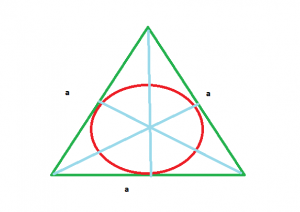Open in App
Not now

# Area of circle which is inscribed in equilateral triangle

• Last Updated : 27 Aug, 2022

Given here is an equilateral triangle with side length a, the task is to find the area of the circle inscribed in that equilateral triangle.
Examples:

```Input : a = 4
Output : 4.1887902047863905

Input : a = 10
Output : 26.1799387799```Approach:

Area of equilateral triangle =Semi perimeter of equilateral triangle = (a + a + a) / 2
Radius of inscribed circle r = Area of equilateral triangle / Semi perimeter of equilateral triangle
==Area of circle = PI*(r*r) =

```*** QuickLaTeX cannot compile formula:

*** Error message:
Error: Nothing to show, formula is empty
```

Below is the implementation of above approach:

## C++

 `// C++ program to find the area ` `// of circle which is inscribed` `// in equilateral triangle ` `# include` `# define PI 3.14` `using` `namespace` `std;`   `// Function return the area of circle ` `// inscribed in equilateral triangle ` `float` `circle_inscribed(``int` `a)` `{` `    ``return` `PI * (a * a) / 12;` `}`   `// Driver code ` `int` `main()` `{` `    ``int` `a = 4;`   `    ``cout << circle_inscribed(a);` `    ``return` `0;` `}`   `// This code is contributed ` `// by Mahadev99`

## Java

 `// Java program to find the area ` `// of circle which is inscribed` `// in equilateral triangle ` `import` `java.io.*;`   `class` `GFG ` `{`   `static` `double` `PI = ``3.14``;`   `// Function return the area of circle ` `// inscribed in equilateral triangle ` `static` `double` `circle_inscribed(``int` `a)` `{` `    ``return` `PI * (a * a) / ``12``;` `}`   `// Driver code ` `public` `static` `void` `main (String[] args) ` `{` `    ``int` `a = ``4``;`   `    ``System.out.println(circle_inscribed(a));` `}` `}`   `// This code is contributed by anuj_67`

## Python3

 `# Python3 program to find the area of circle` `# which is inscribed in equilateral triangle`   `# import math library for pi value` `from` `math ``import` `pi`   `# Function return the area of circle` `# inscribed in equilateral triangle` `def` `circle_inscribed(a):` `    ``return` `pi``*``(a ``*` `a) ``/` `12`   `# Driver code` `a ``=` `4` `print``(circle_inscribed(a))`

## C#

 `// C# program to find the area ` `// of circle which is inscribed` `// in equilateral triangle ` `using` `System;`   `class` `GFG ` `{` `static` `double` `PI = 3.14;`   `// Function return the area of circle ` `// inscribed in equilateral triangle ` `static` `double` `circle_inscribed(``int` `a)` `{` `    ``return` `PI * (a * a) / 12;` `}`   `// Driver code ` `public` `static` `void` `Main ()` `{` `    ``int` `a = 4;`   `    ``Console.WriteLine( circle_inscribed(a));` `}` `}`   `// This code is contributed ` `// by inder_verma`

## PHP

 `

## Javascript

 ``

Output

`4.18667`

Time complexity: O(1), since there is no loop or recursion.

Auxiliary Space: O(1), since no extra space has been taken.

My Personal Notes arrow_drop_up
Related Articles